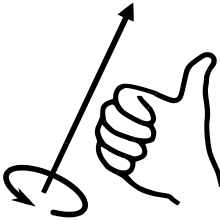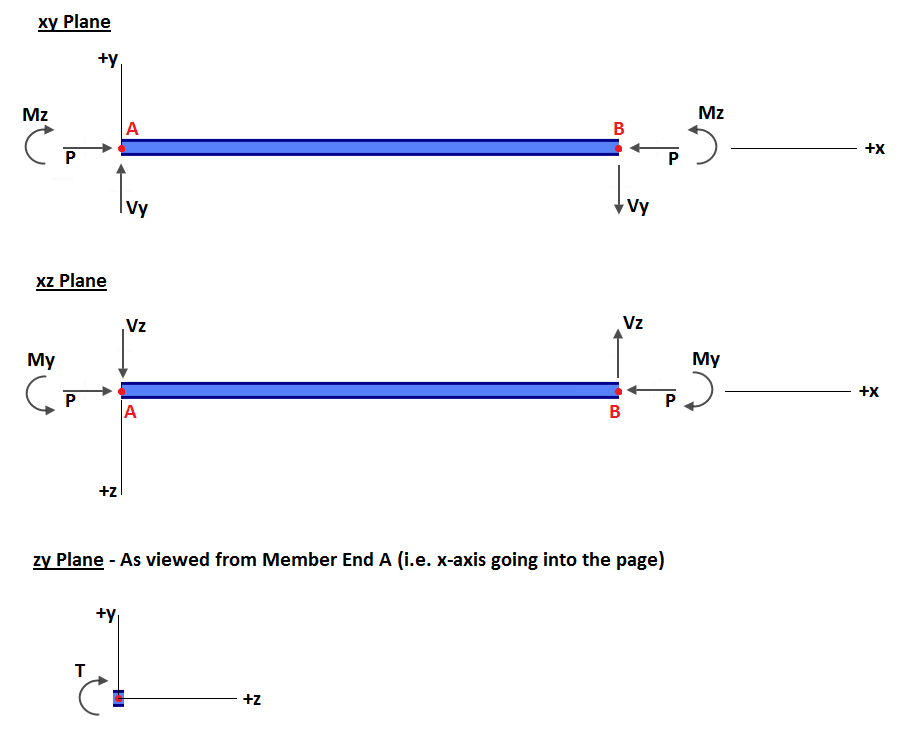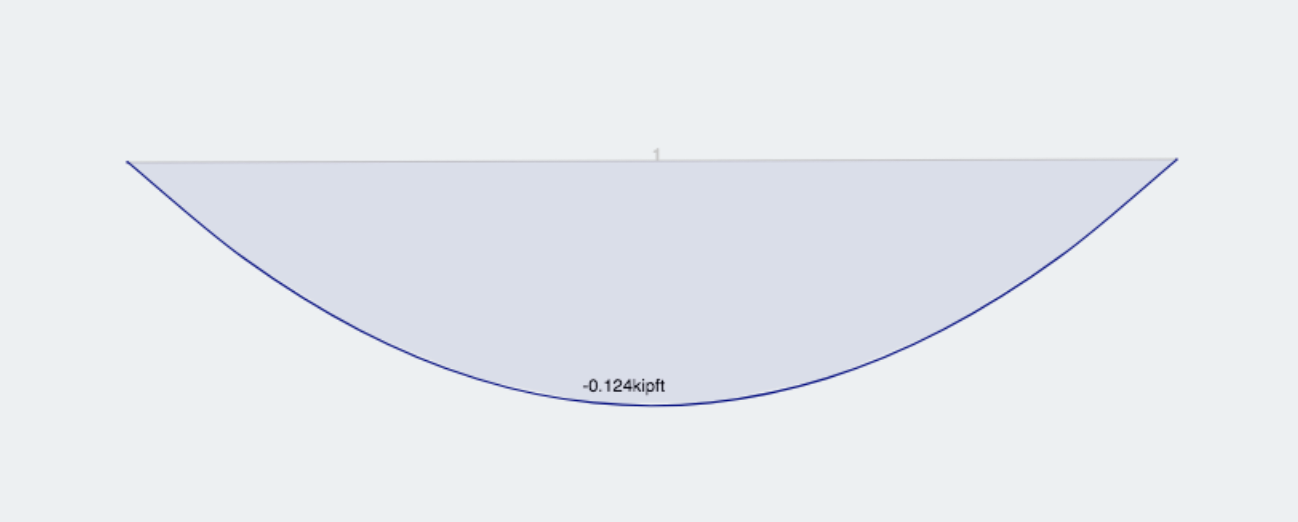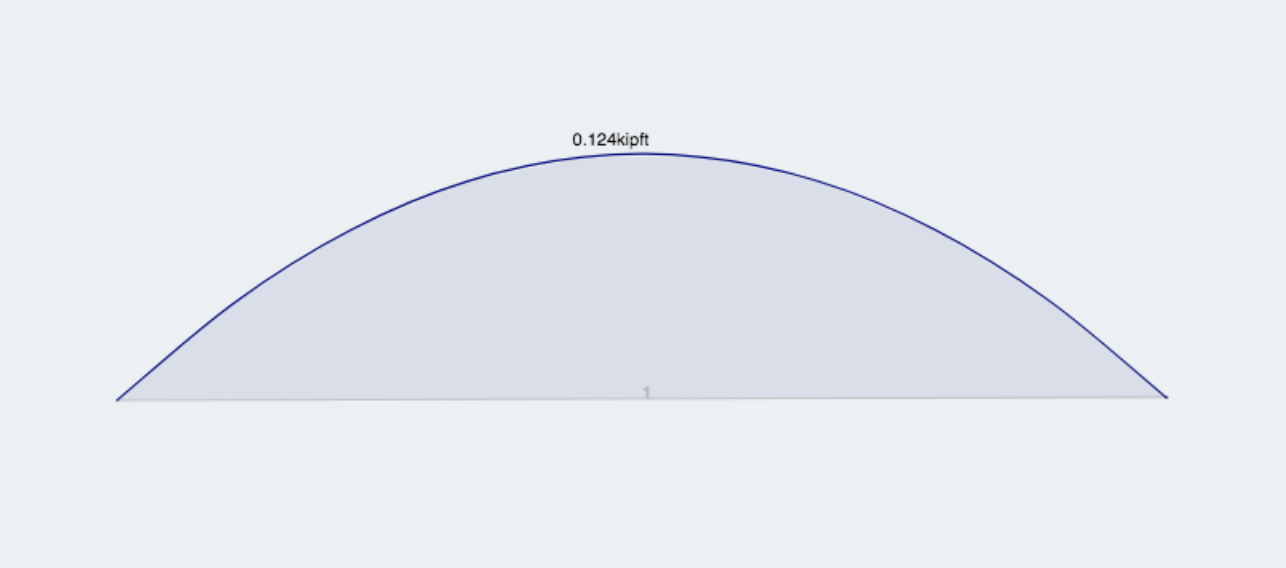SkyCiv文档

1. SkyCiv结构3D
2. 后期处理
3. 标志约定

# 标志约定

## Moment Sign Conventions

Applied loads, 位移, and reactions are positive when they act along or about the positive axis direction. For rotations (like moments) the positive direction is with respect to the RIGHT-HAND RULE:Be wary of the axes which are used to define loads. 默认, loads are along the global axes however distributed loads can be defined with respect to the local member axis. 相似地, plate pressures can be defined globally or locally to the plate. 也, remember that the local axes of members and plates can be viewed and toggled from the 可见性设置.

## Member Actions• P = Axial Force
• T = Torsional Force (即. moment about member’s x-axis)
• Vy = Shear Force in member’s y-axis
• Vz = Shear Force in member’s z-axis
• My = Bending Moment in member’s y-axis
• Mz = Bending Moment in member’s z-axis

The diagram above can be summarized as follows:

• Positive Axial Force (P): 压缩
• Positive Moment (中号): Compression on the positive axis side of the member
• Positive Shear (V): Node A of the member translates in the positive axis with respect to Node B.
• Positive Torsion (Ť): Node B rotates clockwise when viewed from the Node A end. Or Node A of the member rotates counter-clockwise when viewed from the Node B end. Both instances conform to the right-hand rule.

## Simple ExampleIf the ‘Reverse BMD Signsetting is toggled on, the exact same model would produce the following bending moment diagram:Coming soon